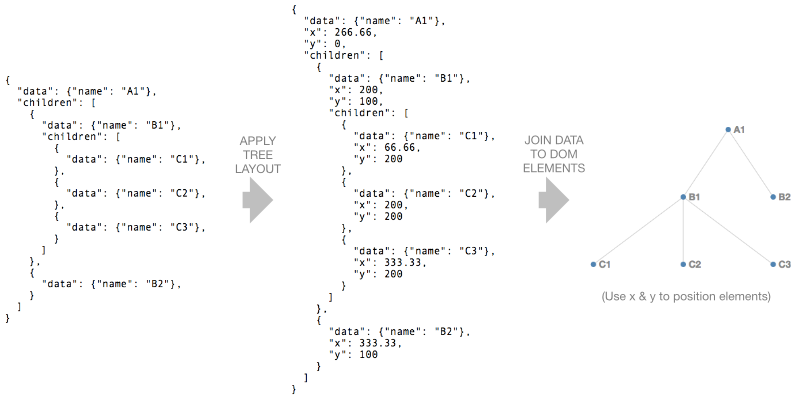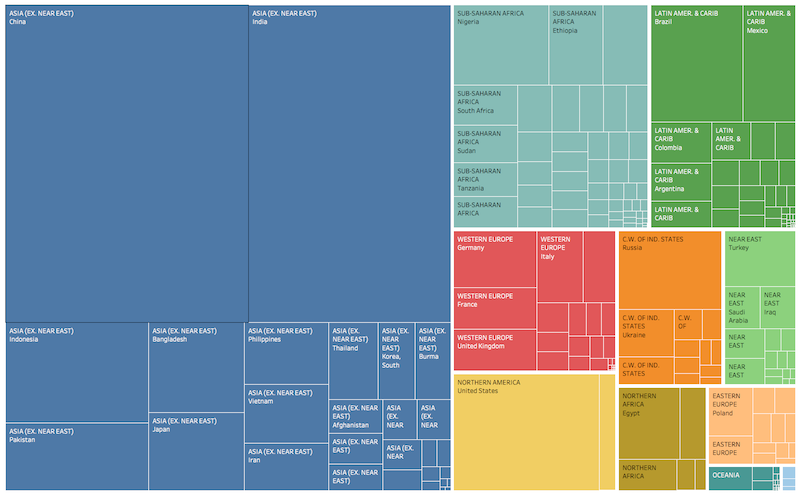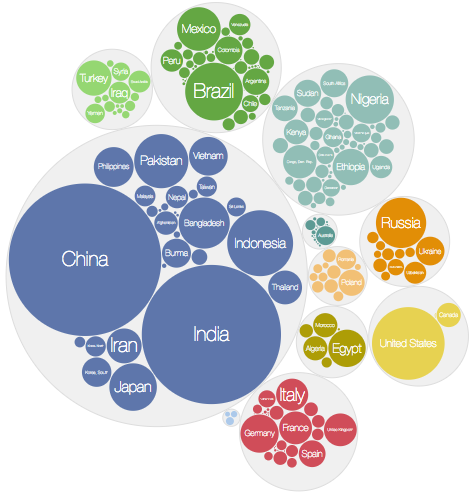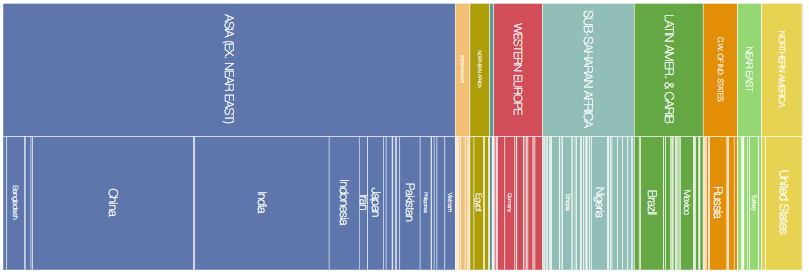## Layouts

packed circles:

and network graphs:

In essence a layout is just a JavaScript function that takes your data as input and adds visual variables such as position and size to it.

For example the tree layout takes a hierarchical data structure and adds `x` and `y` values to each node such that the nodes form a tree-like shape:D3 has a number of hierarchy layouts for dealing with hierarchical (or tree) data as well as a chord layout (for network flows) and a general purpose force layout (physics-based simulation). We’ll cover the force layout in a separate chapter.

(It’s also possible to create your own layouts. For example a simple function that adds positional information to an array of data can be considered a layout.)

### Hierarchical layouts

D3 has a number of hierarchical layouts to help with visualising hierarchies (or trees) e.g.

In this section we’ll look at the `tree`, `cluster`, `treemap`, `pack` and `partition` layouts. Note that `treemap`, `pack` and `partition` are designed to lay out hierarchies where the nodes have an associated numeric value (e.g. revenue, population etc.).

D3 version 4 requires the hierarchical data to be in the form of a `d3.hierarchy` object which we’ll cover next.

#### d3.hierarchy

A `d3.hierarchy` object is a data structure that represents a hierarchy. It has a number of functions defined on it for retrieving things like ancestor, descendant and leaf nodes and for computing the path between nodes. It can be created from a nested JavaScript object such as:

Typically you don’t need to operate on the hierarchy object itself but there are some useful functions defined on it such as:

`root.descendants()` returns a flat array of `root`’s descendants and `root.links()` returns a flat array of objects containing all the parent-child links.

More examples of hierarchy functions

We’ll now look at the `tree`, `cluster`, `treemap`, `pack` and `partition` layouts.

#### tree layout

The `tree` layout arranges the nodes of a hierarchy in a tree like arrangement.

We start by creating the tree layout using:

We can configure the tree’s size using `.size`:

We can then call `treeLayout`, passing in our hierarchy object `root`:

This’ll write `x` and `y` values on each node of `root`.

We can now:

• use `root.descendants()` to get an array of all the nodes
• join this array to circles (or any other type of SVG element)
• use `x` and `y` to position the circles

and

• use `root.links()` to get an array of all the links
• join the array to line (or path) elements
• use `x` and `y` of the link’s source and target to position the line

(In the case of `root.links()` each array element is an object containing two properties `source` and `target` which represent the link’s source and target nodes.)

### cluster layout

The `cluster` layout is very similar to the `tree` layout the main difference being all leaf nodes are placed at the same depth.

### treemap layout

Treemaps were invented by Ben Shneiderman to visually represent hierarchies where each item has an associated value.

For example, we can think of country population data as a hierarchy where the first level represents the region and the next level represents each country. A treemap will represent each country as a rectangle (sized proportionally to the population) and group each region together:D3’s `treemap` layout is created using:

As usual we can configure our layout e.g.

Before applying this layout to our hierarchy we must run `.sum()` on the hierarchy. This traverses the tree and sets `.value` on each node to the sum of its children:

Note that we pass an accessor function into `.sum()` to specify which property to sum.

We can now call `treemapLayout`, passing in our hierarchy object:

The layout adds 4 properties `x0`, `x1`, `y0` and `y1` to each node which specify the dimensions of each rectangle in the treemap.

Now we can join our nodes to `rect` elements and update the `x`, `y`, `width` and `height` properties of each `rect`:

If we’d like labels in each rectangle we could join `g` elements to the array and add `rect` and `text` elements to each `g`:

`treemap` layouts can be configured in a number of ways:

• the padding around a node’s children can be set using `.paddingOuter`
• the padding between sibling nodes can be set using `.paddingInner`
• outer and inner padding can be set at the same time using `.padding`
• the outer padding can also be fine tuned using `.paddingTop`, `.paddingBottom`, `.paddingLeft` and `.paddingRight`.

In the example above `paddingTop` is 20 and `paddingInner` is 2.

Treemaps can use different tiling strategies and D3 has several built in (`treemapBinary`, `treemapDice`, `treemapSlice`, `treemapSliceDice`, `treemapSquarify`) and the configuration function `.tile` is used to select one:

`treemapBinary` strives for a balance between horizontal and vertical partitions, `treemapDice` partitions horizontally, `treemapSlice` partitions vertically, `treemapSliceDice` alternates between horizontal and vertical partioning and `treemapSquarify` allows the aspect ratio of the rectangles to be influenced.

The effect of different squarify ratios can be seen here.

### pack layout

The pack layout is similar to the tree layout but circles instead of rectangles are used to represent nodes. In the example below each country is represented by a circle (sized according to population) and the countries are grouped by region.D3’s `pack` layout is created using:

As usual we can configure its size:

As with the `treemap` we must call `.sum()` on the hierarchy object `root` before applying the `pack` layout:

The `pack` layout adds `x`, `y` and `r` (for radius) properties to each node.

Now we can add `circle` elements for each descendant of `root`:

Labels can be added by creating `g` elements for each descendant:

The padding around each circle can be configured using `.padding()`:

### partition layout

The `partition` layout subdivides a rectangular space into a layer for each layer of the hierarchy. Each layer is subdivided for each node in the layer:D3’s `partition` layout is created using:

As usual we can configure its size:

As with the `treemap` we must call `.sum()` on the hierarchy object `root` and before applying the `partition` layout:

The `partition` layout adds `x0`, `x1`, `y0` and `y1` properties to each node.

We can now add `rect` elements for each descendant of `root`:

Padding can be added between nodes using `.padding()`:

If we’d like to change the orientation of the partition layout so that the layers run left to right we can swap `x0` with `y0` and `x1` with `y1` when defining the `rect` elements:

We can also map the `x` dimension into a rotation angle and `y` into a radius to create a sunburst partition:

### chord layout

Chord diagrams visualise links (or flows) between a group of nodes, where each flow has a numeric value. For example, they can show migration flows between countries. (Personally I find them difficult to interpret!)

The data needs to be in the form of an n x n matrix (where n is the number of items):

The first row represents flows from the 1st item to the 1st, 2nd and 3rd items etc.

We create the layout using:

and we configure it using `.padAngle()` (to set the angle between adjacent groups in radians), `.sortGroups()` (to specify the order of the groups), `.sortSubgroups()` (to sort within each group) and `.sortChords()` to determine the z order of the chords.

We apply the layout using:

which returns an array of chords. Each element of the array is an object with `source` and `target` properties. Each `source` and `target` has `startAngle` and `endAngle` properties which will define the shape of each chord.

We use the `ribbon` shape generator which converts the chord properties into path data (see the Shapes chapter for more information on shape generators).